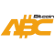Bitcoin ABC  0.27.14 P2P Digital Currency
i2p Namespace Reference

sam

## Classes

struct  Connection
An established connection with another peer. More...

## Typedefs

using Binary = std::vector< uint8_t >
Binary data. More...

## Functions

static std::string SwapBase64 (const std::string &from)
Swap Standard Base64 <-> I2P Base64. More...

static Binary DecodeI2PBase64 (const std::string &i2p_b64)
Decode an I2P-style Base64 string. More...

Derive the .b32.i2p address of an I2P destination (binary). More...

Derive the .b32.i2p address of an I2P destination (I2P-style Base64). More...

## ◆ Binary

 using i2p::Binary = typedef std::vector

Binary data.

Definition at line 26 of file i2p.h.

## ◆ DecodeI2PBase64()

 static Binary i2p::DecodeI2PBase64 ( const std::string & i2p_b64 )
static

Decode an I2P-style Base64 string.

Parameters
 [in] i2p_b64 I2P-style Base64 string.
Returns
decoded `i2p_b64`
Exceptions
 std::runtime_error if decoding fails

Definition at line 69 of file i2p.cpp.

Here is the call graph for this function:
Here is the caller graph for this function:

static

Derive the .b32.i2p address of an I2P destination (I2P-style Base64).

Parameters
 [in] dest I2P destination.
Returns
the address that corresponds to `dest`
Exceptions
 std::runtime_error if conversion fails

Definition at line 108 of file i2p.cpp.

Here is the call graph for this function:
Here is the caller graph for this function:

static

Derive the .b32.i2p address of an I2P destination (binary).

Parameters
 [in] dest I2P destination.
Returns
the address that corresponds to `dest`
Exceptions
 std::runtime_error if conversion fails

Definition at line 86 of file i2p.cpp.

Here is the call graph for this function:
Here is the caller graph for this function:

## ◆ SwapBase64()

 static std::string i2p::SwapBase64 ( const std::string & from )
static

Swap Standard Base64 <-> I2P Base64.

Standard Base64 uses `+` and `/` as last two characters of its alphabet. I2P Base64 uses `-` and `~` respectively. So it is easy to detect in which one is the input and convert to the other.

Parameters
 [in] from Input to convert.
Returns
converted `from`

Definition at line 38 of file i2p.cpp.

Here is the caller graph for this function: